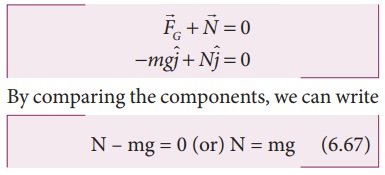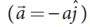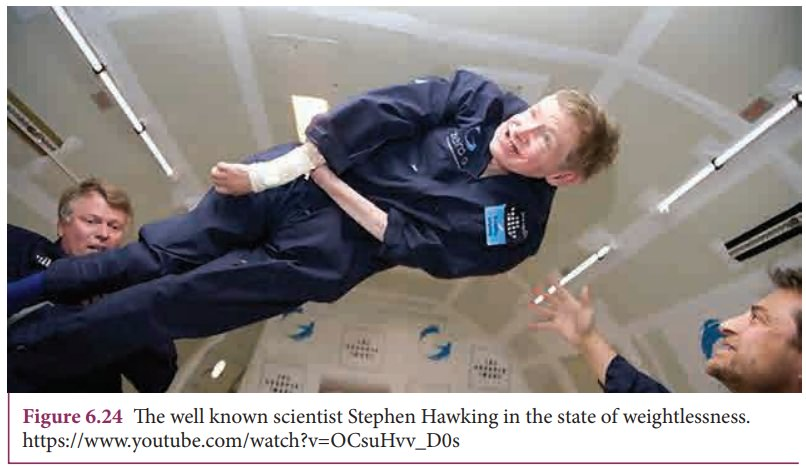Home | | Physics 11th std | Weightlessness Weight of an object - Escape Speed and Orbital Speed

# Weightlessness Weight of an object - Escape Speed and Orbital Speed

Objects on Earth experience the gravitational force of Earth. The gravitational force acting on an object of mass m is mg.

Weightlessness Weight of an object

Objects on Earth experience the gravitational force of Earth. The gravitational force acting on an object of mass m is mg. This force always acts downwards towards the center of the Earth. When we stand on the floor, there are two forces acting on us. One is the gravitational force, acting downwards and the other is the normal force exerted by the floor upwards on us to keep us at rest. The weight of an objectis defined as the downward force whose magnitude W is equal to that of upward force that must be applied to the object to hold it at rest or at constant velocity relative to the earth. The direction of weight is in the direction of gravitational force. So the magnitude of weight of an object is denoted as, W=N=mg. Note that even though magnitude of weight is equal to mg, it is not same as gravitational force acting on the object.

## Apparent weight in elevators

Everyone who used an elevator would have felt a jerk when the elevator takes off or stops. Why does it happen? Understanding the concept of weight is crucial for explaining this effect. Let us consider a man inside an elevator in the following scenarios.

When a man is standing in the elevator, there are two forces acting on him.

1. Gravitational force which acts downward. If we take the vertical direction as positive y direction, the gravitational force acting on the man is2. The normal force exerted by floor on the man which acts vertically upward,## Case (i) When the elevator is at rest

The acceleration of the man is zero. Therefore the net force acting on the man is zero. With respect to inertial frame (ground), applying Newtonâ€™s second law on the man,Since weight, W =N, the apparent weight of the man is equal to his actual weight.

## Case (ii) When the elevator is moving uniformly in the upward or downward direction

In uniform motion (constant velocity), the net force acting on the man is still zero.

Hence, in this case also the apparent weight of the man is equal to his actual weight. It is shown in Figure 6.23(a)## Case (iii) When the elevator is accelerating upwards

If an elevator is moving with upward accelerationwith respect to inertial frame (ground), applying Newtonâ€™s second law on the man,Writing the above equation in terms of unit vector in the vertical direction,Therefore, apparent weight of the man is greater than his actual weight. It is shown in Figure 6.23(b)

## Case (iv) When the elevator is accelerating downwards

If the elevator is moving with downward acceleration, by applying Newtonâ€™s second law on the man, we can writeWriting the above equation in terms of unit vector in the vertical direction,Therefore, apparent weight W = N = m(g-a) of the man is lesser than his actual weight. It is shown in Figure 6.23(c)

## Weightlessness of freely falling bodies

Freely falling objects experience only gravitational force. As they fall freely, they are not in contact with any surface (by neglecting air friction). The normal force acting on the object is zero. The downward acceleration is equal to the acceleration due to the gravity of the Earth. i.e (a = g). From equation (6.69) we get.This is called the state of weightlessness. When the lift falls (when the lift wire cuts) with downward acceleration aâ€†=â€†g, the person inside the elevator is in the state of weightlessness or free fall. It is shown in Figure 6.23(d)When the apple was falling from the tree it was weightless.As soon as it hit Newtonâ€™s head, it gained weight! and Newton gained physics!

## Weightlessness in satellites:

There is a wrong notion that the astronauts in satellites experience no gravitational force because they are far away from the Earth. Actually the Earth satellites that orbit very close to Earth experience only gravitational force. The astronauts inside the satellite also experience the same gravitational force. Because of this, they cannot exert any force on the floor of the satellite. Thus, the floor of the satellite also cannot exert any normal force on the astronaut. Therefore, the astronauts inside a satellite are in the state of weightlessness. Not only the astronauts, but all the objects in the satellite will be in the state of weightlessness which is similar to that of a free fall. It is shown in the Figure 6.24.Study Material, Lecturing Notes, Assignment, Reference, Wiki description explanation, brief detail
11th Physics : UNIT 6 : Gravitation : Weightlessness Weight of an object - Escape Speed and Orbital Speed |